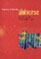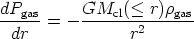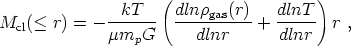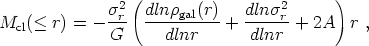© CAMBRIDGE UNIVERSITY PRESS 1999

3.4. The Intracluster Gas: Hydrostatic Equilibrium?

The standard model of clusters assumes that both the gas and the galaxies are in approximate hydrostatic equilibrium with the binding cluster potential (Bahcall and Sarazin 1977; Forman and Jones 1984; Sarazin 1986; Evrard 1990; Bahcall and Lubin 1994). In this model the gas distribution obeys(32)

where Pgas andgas are the gas pressure and density, and Mcl(r) is the total cluster binding mass within a radius r. The cluster mass can thus be represented as(33)

where T is the gas temperature and µmp is the mean particle mass of the gas.

The galaxies in the cluster respond to the same gravitational field, and they satisfy(34)

wherer is the radial velocity dispersion of galaxies in the cluster,gal(r) is the galaxy density profile, and A represents a possible anisotropy in the galaxy velocity distribution [A = 1 - (t /r)2, where t and r represent the tangential and radial velocity components].

The above two relations yield(35)

where thespec parameter, defined by the left side of the above relation, can be determined directly from observations of cluster velocity dispersions and gas temperatures. Thespec parameter represents the ratio of energy per unit mass in the galaxies to that in the gas. Observations of a large sample of clusters yield a mean best-fit value ofspec1 ± 0.1 (Lubin and Bahcall 1993; see also Section 3.5). This suggests that, on average, the gas and galaxies follow each other with comparable energies (2rkT/µmp). The observed mean valuespec1 ± 0.1 is consistent with the value ofdetermined from the right-hand side of therelation (referred to asfit, and determined from the gas and galaxy density profile fits). Usinggas(r)r-2 (Section 3.2) andgal(r)r-2.4 ± 0.2 (Section 2.6), one findsfit0.85 ± 0.1 (for an isothermal distribution) (Bahcall and Lubin 1994). The above consistency supports the assumption that the gas is in an approximate hydrostatic equilibrium with the cluster potential, and suggests that the galaxies and gas approximately trace each other in the clusters.